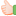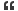# Thread: sizeof() operator

1. ##sizeof() operator

give the o/p for the following code ....also explain how...

int i=10,j;
j=sizeof(++i + ++i);
printf("%d %d",i,j);Reply With Quote

2. ## Re: sizeof() operator

Integer size is 2 bytes. so j carring value 2 and i carrying value 12.Reply With Quote

3. ## Re: sizeof() operator

the result is i=12 and j=2Reply With Quote

4. ##Re: sizeof() operatorOriginally Posted by SriroshikaInteger size is 2 bytes. so j carring value 2 and i carrying value 12.
thanks for the answer...but i carries the same 10 and j carries 2...
test the code please and let me know y the value of i remains the same..Reply With Quote

5. ## Re: sizeof() operatorOriginally Posted by jaiprabukthanks for the answer...but i carries the same 10 and j carries 2...
test the code please and let me know y the value of i remains the same..
how i carry the value 12 please give the detial answerReply With Quote

6. ## Re: sizeof() operator

It depends on size of integer.
/* Assuming size of int as 32 bits */
10 4Reply With Quote

7. ## Re: sizeof() operator

Hi,

A principal use of the sizeof operator is in communication with routines such as storage allocators. A storage-allocation function might accept a size (in bytes)and allocate and return a pointer to void.

Another use of the sizeof operator is to compute the number of elements in an array:
sizeof array / sizeof array

I have not come across any use as belowOriginally Posted by jaiprabukgive the o/p for the following code ....also explain how...

int i=10,j;
j=sizeof(++i + ++i);
printf("%d %d",i,j);
however

generic output is
10 and X(X=One word Length)

10 and 2(on a 16 bit )
10 and 4(on a 32 bit )
10 and 8(on a 64 bit )

Here as we may expect the sizeof has an expression in it and we assume it to be evaluated. But this is not the case as the ISO C standard define the sizeof opeator having constraint as
"The sizeof operator shall not be applied to an expression that has function type or an incomplete type, to the parenthesized name of such a type, or to an expression that designates a bit-field member."

The operand of a sizeof operator is usually not evaluated .

I have referred Committee Draft  Septermber 7, 2007 ISO/IEC 9899:TC3

Regards,
younus saleemReply With Quote

8. ## Re: sizeof() operator

i=12
j=2(dos)
j=4(windows)Reply With Quote

9. ## Re: sizeof() operator

Yes,I would agree to Younus.sizeof() doesn't allow any operation inside it.That's why the o/p should be 10 and 4 or 2 depending on the configuration of the user's m/c.Reply With Quote

10. ## Re: sizeof() operator

4, 8
sizeof operator gives the no of bits it can occupy.Reply With Quote

11.

####Posting Permissions

• You may not post new threads
• You may not post replies
• You may not post attachments
• You may not edit your posts
•